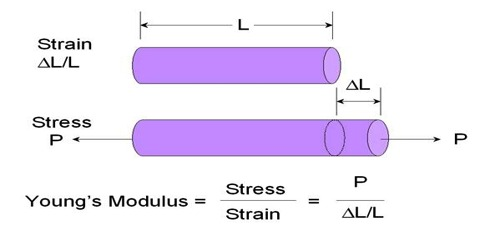# Young’s Modulus

Young’s modulus is a mechanical property that measures the stiffness of a solid material. It defines the relationship between stress and strain in a material in the linear elasticity regime of uniaxial deformation. It is used by engineers to make sure their materials can withstand a sufficient amount of force for the product they are designing. An example of this would be testing a structural engineer would run on the materials being used to build a bridge, which ultimately leads to a weight limit being found which is specific to that bridge.

Young’s modulus – Within the elastic limit ratio of longitudinal stress and longitudinal strain is constant. This constant is called Young’s modulus of the material of the body. It is a measure of the ability of a material to withstand changes in length when under lengthwise tension or compression. It is denoted by Y. The calculation from Young’s modulus depends on the applied force, the type of material and the area of the material.

Young’s modulus, Y = longitudinal stress / longitudinal strain

The stress of the medium relates to the ratio of the applied force with respect to the cross-sectional area. Also, the strain considers the change in length of a material with respect to its original length.Explanation: Hanging from a rigid support one end of a wire of initial length L and radius r and hanging a load at the other end i.e., by applying force F = mg, if the length of the wire increases by l, then,

Longitudinal stress of the wire = F/A and

Longitudinal strain = l/L

So, Young’s modulus, Y = longitudinal stress / longitudinal strain

then, Y = mgL/πr2l

[A = area of cross-section of the wire = πr2]

If in equation Y = FL/Al, A = l unit and l = L; then Y = F.

So, for a wire of unit cross-sectional area if the increase in length is equal to the initial length for the force applied along the length of the wire, then that is called the Young’s modulus.

First, you measure the initial length of the substance. Using a micrometer, you identify the cross-sectional area of the material. Then, with the same micrometer, measure the different diameters of the substance. Next, use various slotted masses to determine the applied force. Physicists would also use Young’s Modulus to test materials and equipment to see how strong they are before using them in high-pressure experiments.

Young’s modulus of steel = 2 x 1011 Nm-2 means that within elastic limit it force of 2 x 1011 N is applied along the length of a steel wire of 1 mm2 cross-sectional area, then the increase of length will be equal to the original length. The quantity stress has the advantage of helping to normalize out the effect of the cross-sectional area for comparison of two samples of the same material.

Dimension of Young’s modulus: [Young’s modulus] = [stress/strain]

or, [Y] = [ML-1T-2]

Unit of Y: Newton/metre2 = [Nm-2]

Uses –

• The Young’s modulus directly Measures the stiffness of the Solid material.
• It defines the relationship between stress and strain in a material.
• It is a measure of the ability of a material to withstand changes in length when under length-wise tension or compression.
• It predicts how much a material sample extends under tension or shortens under compression.
• It is also used in order to predict the deflection that will occur in a statically determinate beam when a load is applied at a point in between the beam’s supports.